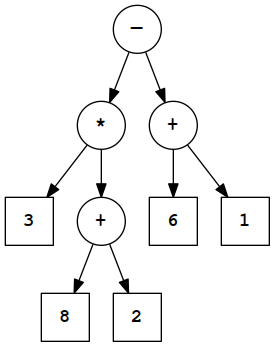# Quiz 4 Solutions

Wed Nov 16

You have up to 25 minutes. You may use a standard calculator if necessary, but no text book, notes, or computer. You may not type the program in to the Python IDLE environment. You should be able to evaluate what the program does on your own. When we say “what is the output,” you should write just the result of the print statements, and label that as output. If you need to write other computations as scratch work, label that as scratch.

1. What is the output of the following Python program?
z1 = 10
z2 = 3
print(z2)
z2 = z2 + z1
if z2 > 5:
print("wow")
print(z1)

Output:

3
wow
10


1. What is the output of the following Python program?
xy = 5
zq = 3
print(xy*2)
if xy > 7:
print("yes")
print(xy + zq)
print("xy")

Output:

10
8
xy


1. Evaluate the following prefix expression. What result does it produce?

(* (+ 3 5) (- 6 2))

⇒ (* 8 (- 6 2))
⇒ (* 8 4)
⇒ 32

2. Evaluate the following postfix expression. What result does it produce?

4 1 2 + * 3 -

Evaluate the operators left to right. Each one refers to the previous two things in the list.

4 1 2 + * 3 -
\___/
4  3  * 3 -
\_____/
12  3 -
\_____/
9

1. Convert the following infix expression to postfix notation:

3 * (8 + 2) - (6 + 1)

You don’t need to produce an explicit tree to convert, but if you do, here’s the correct one:The postfix expression is:

3 8 2 + * 6 1 + -